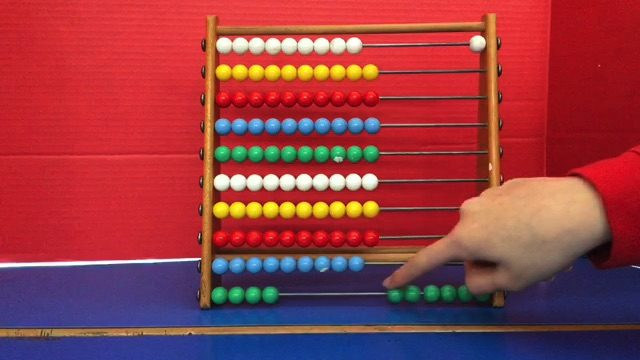# Abacus maths- Zara and EmmaWe learned how to multiply on an abacus with 1 and 2 digit numbers. We watched a video online and practised until we figured out how to do it. After we told the class how to do it then we went round some maths groups. Then planned our own video explaining how to multiply with 1 and 2 digit numbers.

Posted on Categories General, Maths